# Introduction

I had the opportunity to write a detailed explanation of E problem of AtCoder Beginner Contest 177. The result was unexpectedly good, so I will summarize it as an article.

# Consideration

From Problem, the focus can be divided into three in the following order.

1. "GCD (A_i, A_j) = 1 for all 1 ≤ i <j ≤ N" holds.
2. “GCD (A_1 …… A_N) = 1” holds.
3. Neither holds

From now on, we will look at these three in more detail.

## 1. "GCD (A_i, A_j) = 1 for all 1 ≤ i <j ≤ N" holds.

``````N.times do |i|
N.times do |j|
if A[i].gcd(A[j]) != 1 then
is_pairwise_coprime = false
end
end
end
``````

Due to the constraint, \$ 2 ≤ N ≤ 10 ^ 6 \$, so the maximum is \$ O (10 ^ {12}) \$ and the execution time limit of 2 seconds cannot be met.

Note here that `the greatest common divisor of any combination is 1`. This is paraphrased that any combination of integers \$ (A_i, A_j) \$ is relatively prime.

Therefore, all N integers are factorized into prime factors, and if the same prime factors do not appear, this holds, and `pairwise coprime` is output.

## 2. “GCD (A_1 …… A_N) = 1” holds.

This is O (N) even if it is turned honestly, so there is no room for consideration.

``````res = a
a.each do |elm|
res = res.gcd(elm)
end
``````

When 1 does not hold and `res == 1`,` setwise coprime` should be output. Then, when none of 3. is true, `not coprime` is output.

From this, 1 is the main consideration.

# Prime factorization

Ruby has a prime module, which can be factored into prime factors from `Prime.prime_division`. Reference: Play with prime numbers in Ruby (prime module)

This seems to be easy to solve. Submit!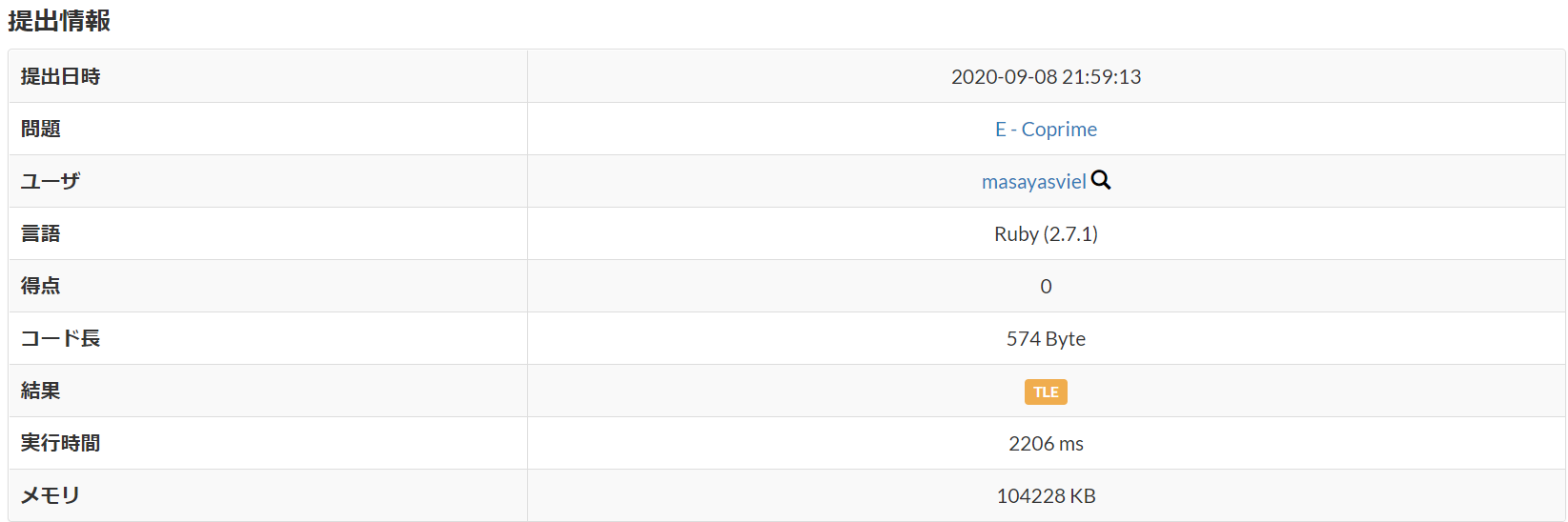Not in time. Then what should we do.

## osa_k method

It is the quickest to have a look at Eratosthenes Sieve and Fast Prime Factorization, but I will explain it in my own way here as well.

This is an algorithm that factores positive integers less than or equal to N (\$ A_ {max} \$ in this case) into prime factors. After preprocessing (\$ O (log log N) ), this process () finds a prime factor of a certain number M. Do O (log M) \$). The amount of calculation is \$ O (log M) \$ from \$ O (log log N + log M) \$. From this maximum value \$ A_ {max} = 10 ^ 6 \$, it becomes about \$ O (log 10 ^ 6) = O (10) \$. Therefore, the amount of calculation to find the prime factors of all N integers is about \$ O (N log A_ {max}) = O (10 ^ 7) \$, which is sufficient.

In summary,

--Prime factorization of positive integers --Perform preprocessing to find the smallest prime factor --High-speed prime factorization using the preprocessed results

Is an algorithm

### Preprocessing

Find the smallest prime factor min_factor for integers (1 ~ N) less than or equal to \$ N = A_ {max} \$. In the application of the Eratosthenes sieve, look at multiples k of i (= 2 ~ \$ \ sqrt {N} \$) in order, and if i is smaller than the value contained in k, enter i.

Example: Take, for example, the maximum value N = 16 of the value to be factored into prime factors. Since \$ \ sqrt {16} = 4 \$, the range of i is 2 ~ 4.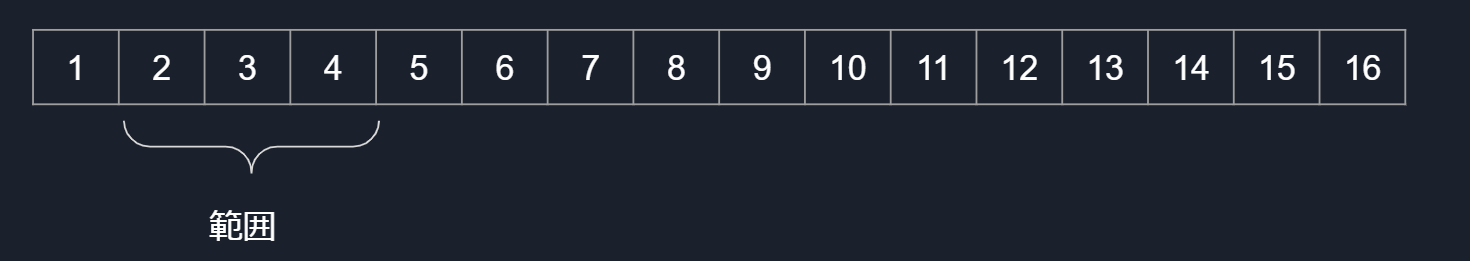Look at i in order and create an array min_factor that stores the smallest prime factors.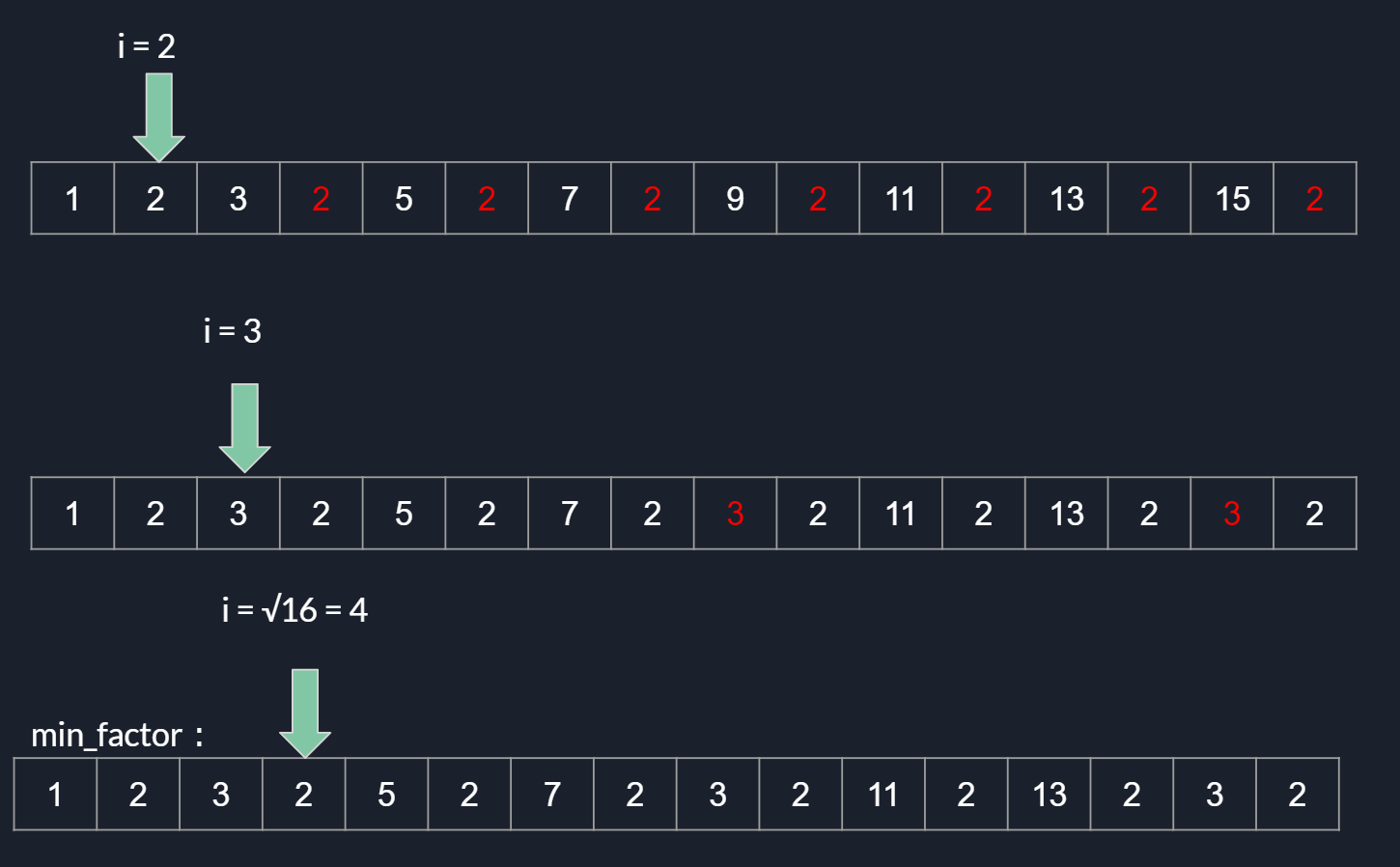The last part is the array min_factor.

### This process

Please refer to the flowchart below.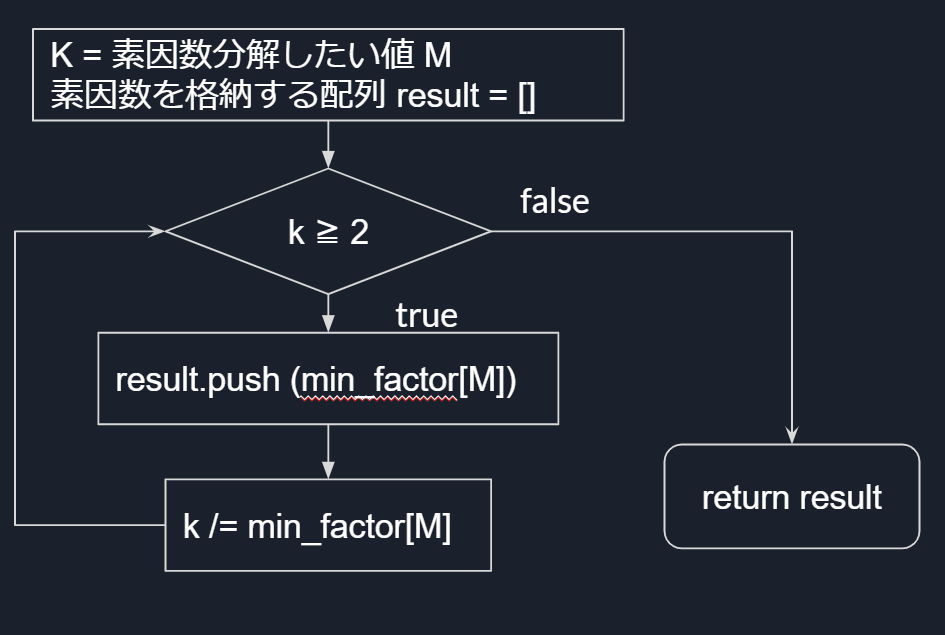The result of prime factorization is stored in result. Since the same prime number is stored multiple times, use ʻuniq` or` set` if you want only prime factors.

When the above example is applied to the above flow, it becomes as shown in the figure below.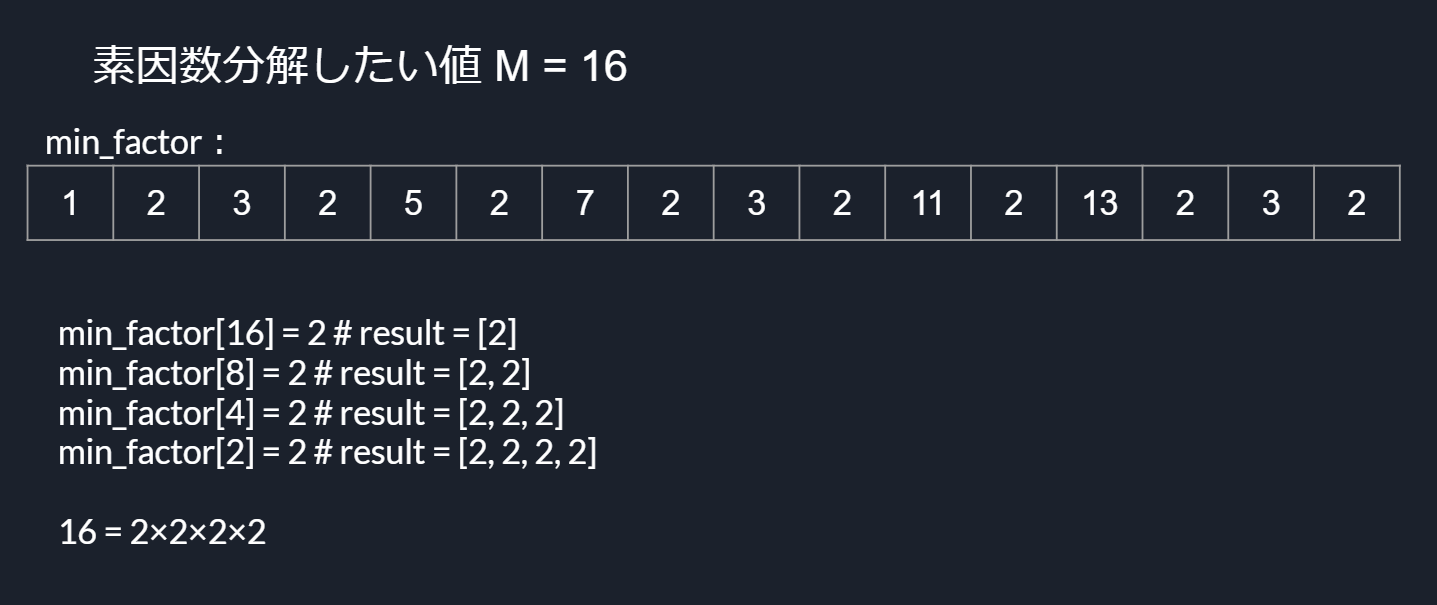Now I can write the code to AC.

# Implementation

``````class Osa_k
# @parm {number} n -Maximum value among the values to be factored into prime factors
def initialize(n)
@min_factor = [*0..n]
i = 2
while i * i <= n do
if @min_factor[i] == i then
j = 2
while i * j <= n do
@min_factor[i*j] = i if @min_factor[i*j] > i
j += 1
end
end
i += 1
end
end

# @parm {number} m -The value you want to factor into
# @return {array} res -Prime factor group of m
def factor(m)
res = []
tmp = m
while tmp > 1 do
res << @min_factor[tmp]
tmp /= @min_factor[tmp]
end
return res.uniq
end
end

MAX = 10 ** 6 + 10 #Maximum value is 10^Because it is 6
n = gets.chomp.to_i
a = gets.chomp.split.map(&:to_i)
osa_k = Osa_k.new(MAX)
res = a
h = Hash.new(0) #Manage whether prime factors have already appeared using associative arrays
is_pairwise_coprime = true #Whether the first condition is met
n.times do |i|
#You don't have to do it when you find that the first condition isn't met
if is_pairwise_coprime then
osa_k.factor(a[i]).each do |num, cnt|
if h.has_key?(num) then
is_pairwise_coprime = false
break
end
h[num] += 1
end
end
res = res.gcd(a[i]) #Check the second condition
end

if is_pairwise_coprime then
puts "pairwise coprime"
elsif res == 1 then
puts "setwise coprime"
else
puts "not coprime"
end
``````

Submission result: https://atcoder.jp/contests/abc177/submissions/16583524

# in conclusion

AC was possible thanks to Eratosthenes sieve and fast prime factorization. I would like to thank you here.